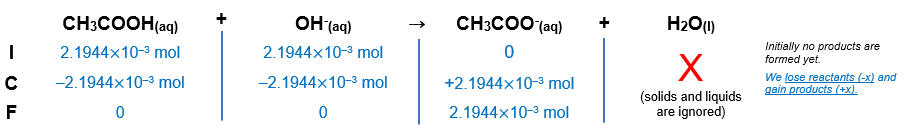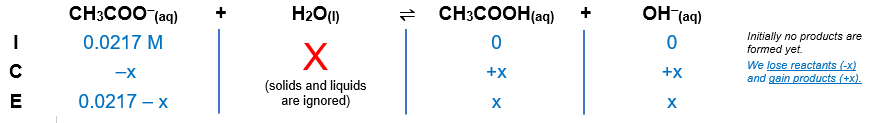# Problem: Find the pH of the equivalence point(s) and the volume (mL) of 0.0372 M NaOH needed to reach the point(s) in titrations of(a) 42.2 mL of 0.0520 M CH 3COOH

###### FREE Expert Solution

NaOH → Na+ + OH

**Na+ → neutral ion

Reaction:      CH3COOH + OH  CH3COO + H2O

Step 1: Calculate the volume (mL) of 0.0372 M NaOH needed to reach the point(s)

molarity (volume) → moles

Recall:

$\overline{){\mathbf{M}}{\mathbf{=}}\frac{\mathbf{mol}}{\mathbf{L}}}$

*convert volumes from mL to L → 1 mL = 10-3 L

at the equivalence point of a titration:

$\overline{){\left(\mathbf{MV}\right)}_{{\mathbf{acid}}}{\mathbf{=}}{\left(\mathbf{MV}\right)}_{{\mathbf{base}}}}$

VNaOH58.99 mL

Step 2. Calculate the initial amounts of acid and base in moles before the reaction happens.

moles CH3COOH = 2.1944×10‒3 mol

moles CH3COOH = moles NaOH

moles NaOH = 2.1944×10‒3 mol

Step 3. Construct an ICF Chart.After the reaction is complete the solution contains:

2.1944×10‒3 mol CH3COO  conjugate base

Step 4. Calculate the new concentration of the species left in the solution.

volume of solution = 0.10119 L

molarity CH3COO = 0.0217 M

Step 5. Calculate pH.

Since only the conjugate base is present after the titration and the conjugate base is a weak base, we will use an ICE chart to find pH.We will need the Kb for the reaction.

Ka CH3COOH = 1.8×105

Ka and Kb are connected by the following equation:

$\overline{){{\mathbf{K}}}_{{\mathbf{w}}}{\mathbf{=}}{{\mathbf{K}}}_{{\mathbf{a}}}{\mathbf{·}}{{\mathbf{K}}}_{{\mathbf{b}}}}$

Where Kw is the autoionization constant of water:
Assuming the reaction is at 25°C:             Kw = 1.0x10-14

Calculate Kb:

$\frac{{\mathbf{K}}_{\mathbf{w}}}{{\mathbf{K}}_{\mathbf{a}}}\mathbf{=}\frac{\overline{){\mathbf{K}}_{\mathbf{a}}}\mathbf{·}{\mathbf{K}}_{\mathbf{b}}}{\overline{){\mathbf{K}}_{\mathbf{a}}}}\phantom{\rule{0ex}{0ex}}\overline{){{\mathbf{K}}}_{{\mathbf{b}}}{\mathbf{=}}\frac{{\mathbf{K}}_{\mathbf{w}}}{{\mathbf{K}}_{\mathbf{a}}}}\phantom{\rule{0ex}{0ex}}{\mathbf{K}}_{\mathbf{b}}\mathbf{=}\frac{\mathbf{1}\mathbf{.}\mathbf{0}\mathbf{×}{\mathbf{10}}^{\mathbf{-}\mathbf{14}}}{\mathbf{1}\mathbf{.}\mathbf{8}\mathbf{×}{\mathbf{10}}^{\mathbf{-}\mathbf{5}}}$

Kb = 5.556x10‒10

Kb is also an equilibrium expression and can be calculated as:

$\overline{){{\mathbf{K}}}_{{\mathbf{b}}}{\mathbf{=}}\frac{\mathbf{products}}{\mathbf{reactants}}}$

Solids and liquids are ignored in equilibrium expressions

$\overline{){{\mathbf{K}}}_{{\mathbf{b}}}{\mathbf{=}}\frac{\left[{\mathbf{CH}}_{\mathbf{3}}\mathbf{COOH}\right]\left[{\mathbf{OH}}^{\mathbf{-}}\right]}{\left[{\mathbf{CH}}_{\mathbf{3}}{\mathbf{COO}}^{\mathbf{-}}\right]}}$

$\mathbf{5}\mathbf{.}\mathbf{556}\mathbf{×}{\mathbf{10}}^{\mathbf{-}\mathbf{10}}\mathbf{=}\frac{\left[\mathbf{x}\right]\left[\mathbf{x}\right]}{\left[\mathbf{0}\mathbf{.}\mathbf{0217}\mathbf{-}\mathbf{x}\right]}$

92% (46 ratings)###### Problem Details

Find the pH of the equivalence point(s) and the volume (mL) of 0.0372 M NaOH needed to reach the point(s) in titrations of

(a) 42.2 mL of 0.0520 M CH 3COOH

Frequently Asked Questions

What scientific concept do you need to know in order to solve this problem?

Our tutors have indicated that to solve this problem you will need to apply the Weak Acid Strong Base Titrations concept. You can view video lessons to learn Weak Acid Strong Base Titrations. Or if you need more Weak Acid Strong Base Titrations practice, you can also practice Weak Acid Strong Base Titrations practice problems.

What textbook is this problem found in?

Our data indicates that this problem or a close variation was asked in Chemistry: The Molecular Nature of Matter and Change - Silberberg 8th Edition. You can also practice Chemistry: The Molecular Nature of Matter and Change - Silberberg 8th Edition practice problems.